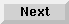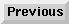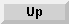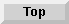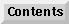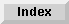7 Additional Extensions to Common Lisp

# 7.4 Floating-point numbers

The numbers that constitute the Lisp type`float` are the floating-point numbers. Common Lisp provides four subtypes of`float`:`short-float`,` single-float`,`double-float`, and`long-float`. These subtypes need not be distinct.

If an implementation of Common Lisp uses only one internal floating-point format, that format is designated as single-float by convention, and any number that is of type` float` is also of type` single-float`,`short-float`,`double-float`, and`long-float`. In Liquid Common Lisp, only one internal format is used. This format is in accordance with the IEEE standard for the representation of 64-bit double-precision floating-point numbers. It includes a sign bit, a 52-bit unsigned mantissa with a hidden bit, and an 11-bit unsigned exponent. The hidden bit in the mantissa is a high-order 1 that is appended to the 52-bit field for normalized floating-point numbers.The exponent is excess-1023; that is, the representation of the exponent is an 11-bit nonnegative integer whose value is 1023 greater than the true exponent value.

Floating-point numbers are represented in radix 2. Negative floating-point numbers use a signed-magnitude representation; that is, there is a distinct negative zero such that the expression`(eql -0.0 0.0)` returns`nil`. The rounding mode is round-to-nearest.

In general, when an operation involves both a rational and a floating-point argument, the rational number is first converted to floating-point format, and then the operation is performed. This conversion process is called floating-point contagion. However, for numerical equality comparisons, the arguments are compared using rational arithmetic to ensure transitivity of the equality (or inequality) relation.

You can make arrays of floating-point numbers that use a packed 32-bit format by specifying the type`single-float` as the argument to the keyword` :element-type` of the Common Lisp function`make-array`. These arrays occupy half of the space required by the default floating-point arrays. Access to elements of single-float arrays causes an automatic conversion from single-float to double-float format. This conversion generally has no adverse performance effects.

Note: The type specifier`(array single-float dimension)` is not equivalent to the type specifier`(array double-float dimension)`. By default, specifying`(array float dimension)` is the same as specifying` (array double-float dimension)`; that is, the elements of the array are double-float quantities. The formats used in floating-point arrays are compatible with C floating-point arrays of the same type.

7.4.1 - Floating-point error conditions
7.4.2 - Floating-point precision
7.4.3 - Floating-point component extraction
7.4.4 - Extreme floating-point numbers
7.4.5 - A known problem
7.4.6 - Reference pages

The Advanced User's Guide - 9 SEP 1996Generated with Harlequin WebMaker